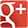•• 1-888-5-HINGES (1-888-544-6437)
or 1-904-285-7575
sales@hanayainc.com

# Torque Calculator1. English Metric
2. lb or kg
3. in or cm
4. in or cm
5. degrees

### How To Calculate The Torque Needed For Your Application

Torque exerted by the panel at any given angle is:

Torque = Weight Of Panel X Length From The Axis Of Rotation To The Center Of Gravity X Cosine Of The Angle

If the weight of the panel is evenly distributed, we can assume that the CG is in the center of the panel. In this case, the length from the axis of rotation to the center of gravity is equal to half the length of the panel.

### Example:

Weight Of Panel = 2 lb

Length Of Panel = 10 in

Length To CG = 5 in (Assuming Weight Is Evenly Distributed)

Minimum Angle Required To Hold The Panel = 30° (If you want the panel to fall at about 30° to closing)

Minimum Torque Required = 2 lb X 5 in X Cos 30° = 8.66 in.lb

Minimum Angle Required To Hold The Panel = 0° (If you want the panel to hold the entire range of motion)

Minimum Torque Required = 2 lb X 5 in X Cos 0° = 10.00 in.lb